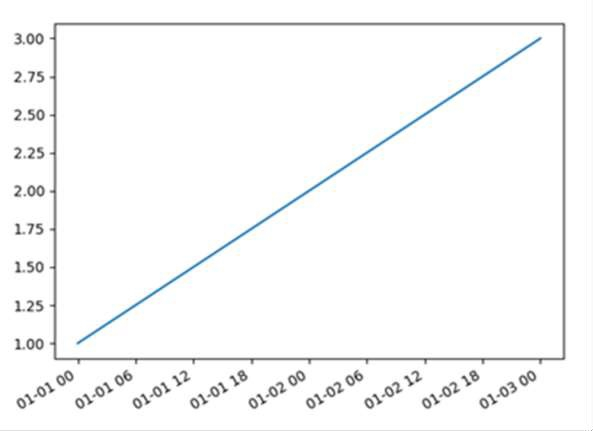# Plotting dates on the X-axis with Python's Matplotlib

MatplotlibPythonServer Side ProgrammingProgramming

Using Pandas, we can create a dataframe and can set the index for datetime. Using gcf().autofmt_xdate(), we will adjust the date on the X-axis.

## Steps

• Make the list of date_time and convert into it in date_time using pd.to_datetime().

• Consider data = [1, 2, 3]

• Instantiate DataFrame() object, i.e., DF.

• Set the DF[‘value’] with data from step 2.

• Set DF.index() using date_time from step 1.

• Now plot the data frame i.e., plt.plot(DF).

• Get the current figure and make it autofmt_xdate().

• Using plt.show() method, show the figure.

## Example

import pandas as pd
import matplotlib.pyplot as plt

date_time = ["2021-01-01", "2021-01-02", "2021-01-03"]
date_time = pd.to_datetime(date_time)
data = [1, 2, 3]

DF = pd.DataFrame()
DF['value'] = data
DF = DF.set_index(date_time)
plt.plot(DF)
plt.gcf().autofmt_xdate()
plt.show()

## Output